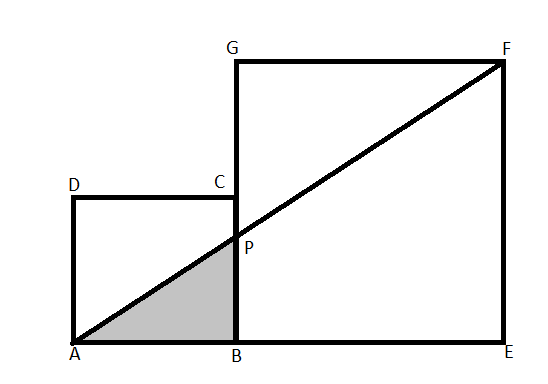QUESTION

# In the figure ABCD and BEFG are two squares with side lengths 4 cm and 10 cm respectively. Find the area of the shaded region (sq. cm).Hint: Length of AB is 4 cm and BC is 10 cm, as given in the question. The coordinate of F is (14,10) and the coordinate of A is (0, 0). Now, we consider A to be the origin of equation AF. By using this, we calculate the value of x and y which are the coordinates of P. Now we can easily calculate the area of triangle APB.

According to the problem statement, the length of AB = 4cm and the length of BC = 10cm.
By using the details of the side of the square, we evaluate the coordinates of F as x = 10+4 = 14 and y = 10 so it can be represented as (14,10).
Consider A to be the origin, the coordinate of A is (0,0).
Now, the equation of line AF:
So, the general equation of line when intercept is zero is: y = mx
Here m is defined as: $m=\dfrac{{{y}_{2}}-{{y}_{1}}}{{{x}_{2}}-{{x}_{1}}}$
Putting the values in slope formula: y2 = 14, y1 = 0, x2 =10 and x1 = 0
\begin{align} & m=\dfrac{14-0}{10-0} \\ & m=\dfrac{7}{5} \\ \end{align}
Putting the value of m is $\dfrac{y}{x}$.
So,
\begin{align} & \dfrac{y}{x}=\dfrac{7}{5} \\ & y=\dfrac{7}{5}x \\ \end{align}
Putting the value of x = 4 cm, we get y $=\dfrac{28}{5}$ cm. They are the coordinates of P.
$P\left( 4,\dfrac{28}{5} \right)$
Now, the area of $\Delta APB$.
Area$=\dfrac{1}{2}\times base\times height$.
Area$=\dfrac{1}{2}\times AB\times BP$.
Here, AB = 4cm, BP $=\dfrac{28}{5}$cm.
Area of $\Delta APB=\dfrac{1}{2}\times 4\times \dfrac{28}{5}$
Area of $\Delta APB=11.2c{{m}^{2}}$.
Hence, the area of the shaded region which is $\Delta APB=11.2c{{m}^{2}}$.

Note: This question can be alternatively solved by using the property of similar triangles. Since the triangle APB and AFE are similar, so the ratio of side PB and FE is proportional to the ratio of side AB and AE. As we know all the values other than PB, so it can be evaluated.